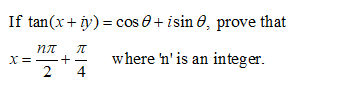If tan(x + y)-cos θ + i sin θ, prove that+즈x = _where n' is an integer

Question

Please solve a question on hyperbolic functions.help_outlineImage TranscriptioncloseIf tan(x + y)-cos θ + i sin θ, prove that +즈 x = _ where n' is an integer fullscreen
Step 1

Let us first find x + iy and its complex conjugate x – iy. Complex conjugate can be found by replacing i with –i in the given complex expression.

Step 2

Adding (x + iy) and (x – iy) equations will cancel iy and will give expression for ...

Want to see the full answer?

See Solution

Want to see this answer and more?

Our solutions are written by experts, many with advanced degrees, and available 24/7

See Solution
Tagged in

Trigonometry